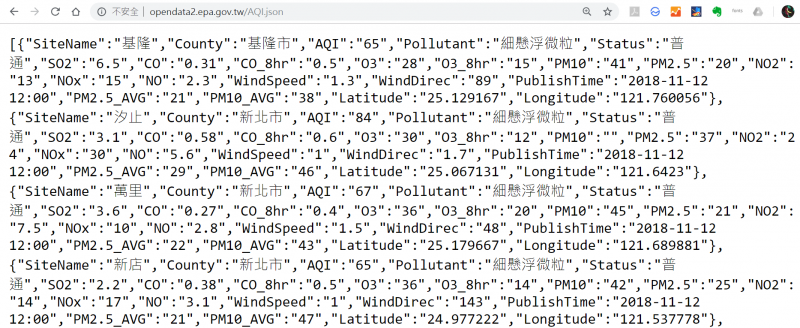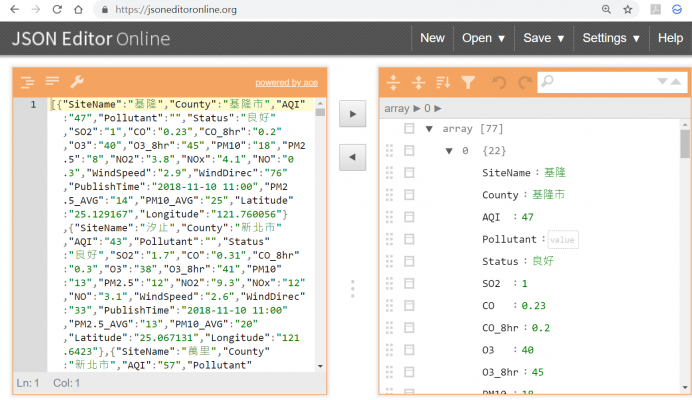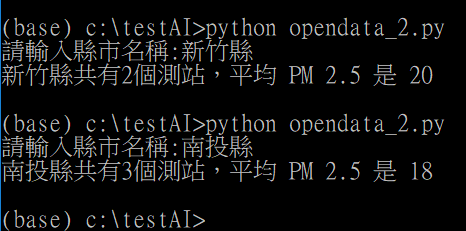# 簡易opendata – PM2.5 查詢source code:

easy example of MS Cognitive service - Face API
``#!/usr/bin/env python3# -*- coding: UTF-8 -*-import urllib, jsonfrom urllib import requests = 0k = 0url = "http://opendata2.epa.gov.tw/AQI.json"response = request.urlopen(url)content = response.read()content = content.decode('utf-8')data_list = json.loads(content)c = input("請輸入縣市名稱:")for i in range(0,81):  if c == data_list[i]["County"]:    s = s + int(data_list[i]["PM2.5"])    k=k+1if k == 0:  print("查無資料")else:  print (c + "共有" + str(k) + "個測站，平均 PM 2.5 是 " + str(round(s/k)))``